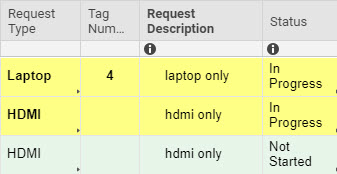# Formula Count Help

May I please get assistance with a formula? I am trying to do a formula that references another sheet (Loaner Sign Out Sheet), which calculates a total from that onto my Request Metrics sheet. Please see attached. I would like a total count for Request Type "HDMI" only if the Status states "In Progress." If it's not in progress I do not want it to count towards the total.

In the example attached the total should = 1 since there's only one HDMI in a "In Progress" status.

Thank you.Tags:

• This is the layout, but you'll need to use the "reference other sheet" when creating the formula to pull the results. Referencing another sheet will put your ranges between { } brackets.

=COUNTIFS({Request Type Range}, ="HDMI", {Status Range}, ="In Progress")

• One thing to keep in mind...

When using specific values (text, number, special character, or a combination thereof) you do not need the operator.

Nic's formula is not wrong. I am just pointing out a little quirk with these types of formulas.

=COUNTIFS({Request Type Range}, = "HDMI", {Status Range}, = "In Progress")

works exactly the same as

=COUNTIFS({Request Type Range}, "HDMI", {Status Range}, "In Progress")

which also works exactly the same as

=COUNTIFS({Request Type Range@}, @cell = "HDMI", {Status Range@}, @cell = "In Progress")

## Help Article Resources

Want to practice working with formulas directly in Smartsheet?

Check out the Formula Handbook template!